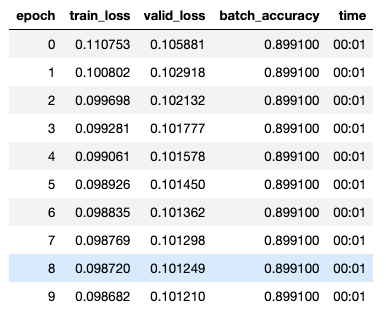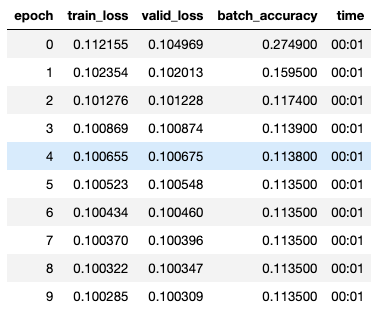# 04_mnist_basics 0-9 Bug

Hi Folks,

I have spent too many hours trying to get past my bug. I am trying this hw assignment using the learner method, simple_net, and batch_accuracy. My problem is that my batch_accuracy is flat (0.89910) and my network give bad results when tested on a few samples. Can you please help find my error? Is something setup incorrectly? Maybe my indices are wrong? How can I “reset” my learner?

(So sorry if this is the wrong category)

Notes:

• train_x and train_y both look good (60k rows 28*28 columns and 60k rows 10 columns)
• the resnet-18 and cross entropy method does work (98% accuracy AND validated on a few pictures)

Here is some code to chew on for the method I am developing and where I need help:

``````def init_params(size, std=1.0): return (torch.randn(size)*std).requires_grad_()

def mnist_loss(predictions, targets):
predictions = predictions.sigmoid()

simple_net = nn.Sequential(
nn.Linear(28*28,30),
nn.ReLU(),
nn.Linear(30,10),
)

def batch_accuracy(xb, yb):
preds = xb.sigmoid()
correct = (preds>0.5) == yb
return correct.float().mean()

dset = list(zip(train_x,train_y))
valid_dset = list(zip(valid_x,valid_y))

xb,yb = first(dl)

w1 = init_params((28*28,30))
b1 = init_params(30)
w2 = init_params((30,10))
b2 = init_params(10)
params = w1,b1,w2,b2

learn = Learner(dls, simple_net, opt_func=SGD,
loss_func=mnist_loss, metrics=batch_accuracy)
learn.fit(10,0.1)``````

Here is my test code for completeness:

``````for i in range (10):

f=pick_a_file(i);
targetTensors = tensor(Image.open(f)).float()/255
targetTensorsv=targetTensors.view(-1,28*28);
learn.model.eval()
res=learn.model(targetTensorsv)
print(tail, res)``````

No improvement of batch_accuracy is a clear indication that something is wrong.

Perhaps what’s wrong is my exceptions?What is the input going into batch_accuracy? Print xb, yb to see.

``````print(xb.shape,yb.shape)
torch.Size([256, 784]) torch.Size([256, 10])
``````

xb looks like this:

``````tensor([[0., 0., 0.,  ..., 0., 0., 0.],
[0., 0., 0.,  ..., 0., 0., 0.],
[0., 0., 0.,  ..., 0., 0., 0.],
[0., 0., 0.,  ..., 0., 0., 0.],
[0., 0., 0.,  ..., 0., 0., 0.]])
``````

yb looks like this:

`````` tensor([[1, 0, 0, 0, 0, 0, 0, 0, 0, 0],
[1, 0, 0, 0, 0, 0, 0, 0, 0, 0],
[1, 0, 0, 0, 0, 0, 0, 0, 0, 0],
[1, 0, 0, 0, 0, 0, 0, 0, 0, 0],
[1, 0, 0, 0, 0, 0, 0, 0, 0, 0]])
``````

I think I know the issue. With a batch size of 256, I need to shuffle my rows. Otherwise, my batches are not varied enough. yb shows this.

Do you think so? (I will try to figure out how to shuffle in the meantime)

Thanks!
Andrew

Here is some more data, in the middle of the first few rows:

``````xb[0:5,210:215] looks like this:
tensor([[0.9882, 0.9922, 0.9882, 0.7922, 0.3294],
[0.9961, 0.6824, 0.2627, 0.1294, 0.7843],
[0.9843, 0.9922, 0.9843, 0.9922, 0.9843],
[0.0000, 0.0000, 0.7490, 1.0000, 1.0000],
[0.9843, 0.9843, 0.9961, 0.9922, 0.2039]])
yb looks like this:
tensor([[1, 0, 0, 0, 0, 0, 0, 0, 0, 0],
[1, 0, 0, 0, 0, 0, 0, 0, 0, 0],
[1, 0, 0, 0, 0, 0, 0, 0, 0, 0],
[1, 0, 0, 0, 0, 0, 0, 0, 0, 0],
[1, 0, 0, 0, 0, 0, 0, 0, 0, 0]])
``````

But INSIDE batch_accuracy function, the data looks weird to me (or maybe a symptom of the error):

``````torch.Size([256, 10]) torch.Size([256, 10])
xb[0:5,:] looks like this:
tensor([[-15.4484, -13.9584, -15.1964, -15.9966, -15.4445, -14.9287, -15.1861, -15.2156, -15.6931, -15.6482],
[-18.1464, -16.1115, -17.5937, -18.3549, -17.8356, -17.3503, -17.6006, -17.5466, -18.2691, -18.0694],
[-12.1793, -10.7864, -12.1959, -12.4902, -12.2340, -11.9365, -12.1230, -12.0399, -12.4235, -12.3694],
[-14.9860, -13.6031, -14.9265, -15.5899, -14.9860, -14.5910, -14.8117, -14.8113, -15.2566, -15.1400],
[-14.8813, -13.7318, -14.8832, -15.5768, -14.9929, -14.5114, -14.7468, -14.7860, -15.2780, -15.0961]])
yb looks like this:
tensor([[1, 0, 0, 0, 0, 0, 0, 0, 0, 0],
[1, 0, 0, 0, 0, 0, 0, 0, 0, 0],
[1, 0, 0, 0, 0, 0, 0, 0, 0, 0],
[1, 0, 0, 0, 0, 0, 0, 0, 0, 0],
[1, 0, 0, 0, 0, 0, 0, 0, 0, 0]])``````

xb looks fine, sigmoid hasn’t been applied yet. In fact, the sigmoid can probably be skipped if you want (save computation). For each row in xb, you have to find the index of the maximum value and compared that with the index of yb where the 1 occurs (also maximum value).

return (torch.argmax(xb, dim=1) == torch.argmax(yb, dim=1)).float().mean()

might work.

@nghiaho12 , unfortunately that’s not the issue. batch_accuracy does change (at least). But there is something else fundamentally wrong here. I just can’t seem to find it.I’m pretty sure this can be accomplished to some reasonable performance level. I must be doing something basic incorrectly.

Maybe you can share the notebook?

@nghiaho12 , yep. It’s here:
My Notebook

Here are some suggestions. Since your network is meant to classify 10 digits, I’d change the network definition to

``````simple_net = nn.Sequential(
nn.Linear(28*28,30),
nn.ReLU(),
nn.Linear(30,10),
nn.Softmax(dim=1)
)
``````

This will ensure the predicted vector sums to 1. This will be useful for the loss function.

Your mnist_loss can be simplified to

``````def mnist_loss(predictions, targets):
``````

The softmax removes the need for the explicit sigmoid call you had originally.

Change the batch accuracy to

``````def batch_accuracy(xb, yb):
correct = torch.argmax(xb, dim=1) == torch.argmax(yb, dim=1)
return correct.float().mean()
``````

before calling learn.fit(…) I would call

``````learn.lr_find()
``````

to see what’s a good learning rate. I tried a learning of 1.0 and it seems to work with your example.

1 Like

OK, thanks for the help. The Softmax function is pretty slick and a nice way to keep things inbounds and save resources in other areas of the code. After that, I can see changes to ensure proper “columning” to ensure things are ok. These are the primary fixes, thanks!

I remember the learning rate finder and am not new to that one. The picture looks a little weird for me. I accept that 1.0 is better than 1e-4 which will take time. Here is that pic and a nice look a the accuracy moving around:

In the end, with one net, this is what I see on some images. Not perfect but pretty good:

for i in range (10):

``````f=pick_a_file(i);
targetTensors = tensor(Image.open(f)).float()/255
targetTensorsv=targetTensors.view(-1,28*28);
learn.model.eval()
res=learn.model(targetTensorsv)
#print(torch.argmax(res))
buf = "%s" % torch.argmax(res);
print("Truth:", head[-1],' Detected:', buf, "Filename:", tail)

Truth: 0  Detected: 0 Filename: 1001.png
Truth: 1  Detected: 1 Filename: 1004.png
Truth: 2  Detected: 2 Filename: 1002.png
Truth: 3  Detected: 3 Filename: 1020.png
Truth: 4  Detected: 9 Filename: 1010.png
Truth: 5  Detected: 3 Filename: 1003.png
Truth: 6  Detected: 6 Filename: 100.png
Truth: 7  Detected: 7 Filename: 0.png
Truth: 8  Detected: 9 Filename: 1007.png
Truth: 9  Detected: 9 Filename: 1000.png
``````

I am happy now, can move to ch5, and can sleep at night. Thank you!

Small note for folks wandering into this. From Ch5:

Our advice is to pick either:

• One order of magnitude less than where the minimum loss was achieved (i.e., the minimum divided by 10)
• The last point where the loss was clearly decreasing